Oscillations

# Simple Harmonic Motion

### Learning Objectives

By the end of this section, you will be able to:

• Define the terms period and frequency
• List the characteristics of simple harmonic motion
• Explain the concept of phase shift
• Write the equations of motion for the system of a mass and spring undergoing simple harmonic motion
• Describe the motion of a mass oscillating on a vertical spring

When you pluck a guitar string, the resulting sound has a steady tone and lasts a long time ((Figure)). The string vibrates around an equilibrium position, and one oscillation is completed when the string starts from the initial position, travels to one of the extreme positions, then to the other extreme position, and returns to its initial position. We define periodic motion to be any motion that repeats itself at regular time intervals, such as exhibited by the guitar string or by a child swinging on a swing. In this section, we study the basic characteristics of oscillations and their mathematical description.

When a guitar string is plucked, the string oscillates up and down in periodic motion. The vibrating string causes the surrounding air molecules to oscillate, producing sound waves. (credit: Yutaka Tsutano)### Period and Frequency in Oscillations

In the absence of friction, the time to complete one oscillation remains constant and is called the period (T). Its units are usually seconds, but may be any convenient unit of time. The word ‘period’ refers to the time for some event whether repetitive or not, but in this chapter, we shall deal primarily in periodic motion, which is by definition repetitive.

A concept closely related to period is the frequency of an event. Frequency (f) is defined to be the number of events per unit time. For periodic motion, frequency is the number of oscillations per unit time. The relationship between frequency and period is

$f=\frac{1}{T}.$

The SI unit for frequency is the hertz (Hz) and is defined as one cycle per second:

$1\phantom{\rule{0.2em}{0ex}}\text{Hz}=1\frac{\text{cycle}}{\text{sec}}\phantom{\rule{0.5em}{0ex}}\text{or}\phantom{\rule{0.5em}{0ex}}1\phantom{\rule{0.2em}{0ex}}\text{Hz}=\frac{1}{\text{s}}=1\phantom{\rule{0.2em}{0ex}}{\text{s}}^{-1}.$

A cycle is one complete oscillation.

Determining the Frequency of Medical Ultrasound
Ultrasound machines are used by medical professionals to make images for examining internal organs of the body. An ultrasound machine emits high-frequency sound waves, which reflect off the organs, and a computer receives the waves, using them to create a picture. We can use the formulas presented in this module to determine the frequency, based on what we know about oscillations. Consider a medical imaging device that produces ultrasound by oscillating with a period of $0.400\phantom{\rule{0.2em}{0ex}}\mu \text{s}$. What is the frequency of this oscillation?

Strategy
The period (T) is given and we are asked to find frequency (f).

Solution
Substitute $0.400\phantom{\rule{0.2em}{0ex}}\mu \text{s}$ for T in $f=\frac{1}{T}$:

$f=\frac{1}{T}=\frac{1}{0.400\phantom{\rule{0.2em}{0ex}}×\phantom{\rule{0.2em}{0ex}}{10}^{-6}\phantom{\rule{0.2em}{0ex}}\text{s}}.$

Solve to find

$f=2.50\phantom{\rule{0.2em}{0ex}}×\phantom{\rule{0.2em}{0ex}}{10}^{6}\phantom{\rule{0.2em}{0ex}}\text{Hz}\text{.}$

Significance
This frequency of sound is much higher than the highest frequency that humans can hear (the range of human hearing is 20 Hz to 20,000 Hz); therefore, it is called ultrasound. Appropriate oscillations at this frequency generate ultrasound used for noninvasive medical diagnoses, such as observations of a fetus in the womb.

### Characteristics of Simple Harmonic Motion

A very common type of periodic motion is called simple harmonic motion (SHM). A system that oscillates with SHM is called a simple harmonic oscillator.

Simple Harmonic Motion

In simple harmonic motion, the acceleration of the system, and therefore the net force, is proportional to the displacement and acts in the opposite direction of the displacement.

A good example of SHM is an object with mass m attached to a spring on a frictionless surface, as shown in (Figure). The object oscillates around the equilibrium position, and the net force on the object is equal to the force provided by the spring. This force obeys Hooke’s law ${F}_{s}=\text{−}kx,$ as discussed in a previous chapter.

If the net force can be described by Hooke’s law and there is no damping (slowing down due to friction or other nonconservative forces), then a simple harmonic oscillator oscillates with equal displacement on either side of the equilibrium position, as shown for an object on a spring in (Figure). The maximum displacement from equilibrium is called the amplitude (A). The units for amplitude and displacement are the same but depend on the type of oscillation. For the object on the spring, the units of amplitude and displacement are meters.

An object attached to a spring sliding on a frictionless surface is an uncomplicated simple harmonic oscillator. In the above set of figures, a mass is attached to a spring and placed on a frictionless table. The other end of the spring is attached to the wall. The position of the mass, when the spring is neither stretched nor compressed, is marked as $x=0$ and is the equilibrium position. (a) The mass is displaced to a position $x=A$ and released from rest. (b) The mass accelerates as it moves in the negative x-direction, reaching a maximum negative velocity at $x=0$. (c) The mass continues to move in the negative x-direction, slowing until it comes to a stop at $x=\text{−}A$. (d) The mass now begins to accelerate in the positive x-direction, reaching a positive maximum velocity at $x=0$. (e) The mass then continues to move in the positive direction until it stops at $x=A$. The mass continues in SHM that has an amplitude A and a period T. The object’s maximum speed occurs as it passes through equilibrium. The stiffer the spring is, the smaller the period T. The greater the mass of the object is, the greater the period T.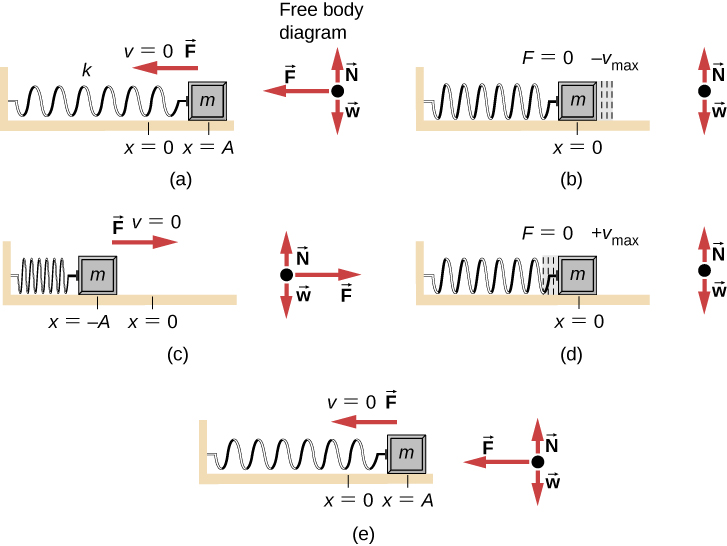What is so significant about SHM? For one thing, the period T and frequency f of a simple harmonic oscillator are independent of amplitude. The string of a guitar, for example, oscillates with the same frequency whether plucked gently or hard.

Two important factors do affect the period of a simple harmonic oscillator. The period is related to how stiff the system is. A very stiff object has a large force constant (k), which causes the system to have a smaller period. For example, you can adjust a diving board’s stiffness—the stiffer it is, the faster it vibrates, and the shorter its period. Period also depends on the mass of the oscillating system. The more massive the system is, the longer the period. For example, a heavy person on a diving board bounces up and down more slowly than a light one. In fact, the mass m and the force constant k are the only factors that affect the period and frequency of SHM. To derive an equation for the period and the frequency, we must first define and analyze the equations of motion. Note that the force constant is sometimes referred to as the spring constant.

### Equations of SHM

Consider a block attached to a spring on a frictionless table ((Figure)). The equilibrium position (the position where the spring is neither stretched nor compressed) is marked as $x=0$. At the equilibrium position, the net force is zero.

A block is attached to a spring and placed on a frictionless table. The equilibrium position, where the spring is neither extended nor compressed, is marked as $x=0.$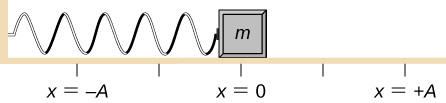Work is done on the block to pull it out to a position of $x=+A,$ and it is then released from rest. The maximum x-position (A) is called the amplitude of the motion. The block begins to oscillate in SHM between $x=+A$ and $x=\text{−}A,$ where A is the amplitude of the motion and T is the period of the oscillation. The period is the time for one oscillation. (Figure) shows the motion of the block as it completes one and a half oscillations after release. (Figure) shows a plot of the position of the block versus time. When the position is plotted versus time, it is clear that the data can be modeled by a cosine function with an amplitude A and a period T. The cosine function $\text{cos}\theta$ repeats every multiple of $2\pi ,$ whereas the motion of the block repeats every period T. However, the function $\text{cos}\left(\frac{2\pi }{T}t\right)$ repeats every integer multiple of the period. The maximum of the cosine function is one, so it is necessary to multiply the cosine function by the amplitude A.

$x\left(t\right)=A\phantom{\rule{0.1em}{0ex}}\text{cos}\left(\frac{2\pi }{T}t\right)=A\phantom{\rule{0.1em}{0ex}}\text{cos}\left(\omega t\right).$

Recall from the chapter on rotation that the angular frequency equals $\omega =\frac{d\theta }{dt}$. In this case, the period is constant, so the angular frequency is defined as $2\pi$ divided by the period, $\omega =\frac{2\pi }{T}$.

A block is attached to one end of a spring and placed on a frictionless table. The other end of the spring is anchored to the wall. The equilibrium position, where the net force equals zero, is marked as $x=0\phantom{\rule{0.2em}{0ex}}\text{m}\text{.}$ Work is done on the block, pulling it out to $x=+A$, and the block is released from rest. The block oscillates between $x=+A$ and $x=\text{−}A$. The force is also shown as a vector.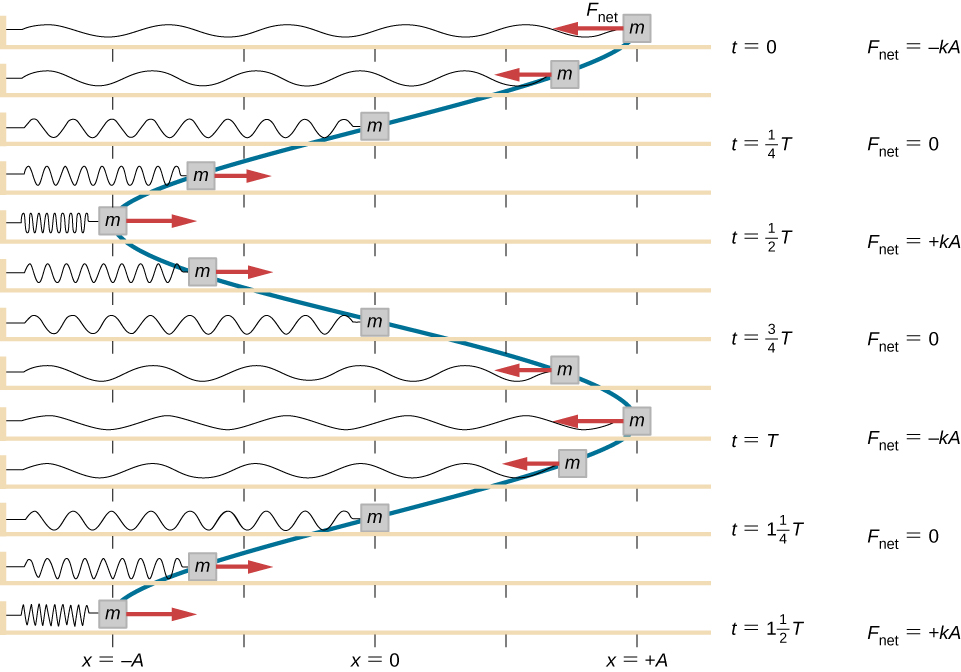A graph of the position of the block shown in (Figure) as a function of time. The position can be modeled as a periodic function, such as a cosine or sine function.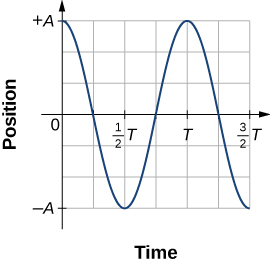The equation for the position as a function of time $x\left(t\right)=A\phantom{\rule{0.1em}{0ex}}\text{cos}\left(\omega t\right)$ is good for modeling data, where the position of the block at the initial time $t=0.00\phantom{\rule{0.2em}{0ex}}\text{s}$ is at the amplitude A and the initial velocity is zero. Often when taking experimental data, the position of the mass at the initial time $t=0.00\phantom{\rule{0.2em}{0ex}}\text{s}$ is not equal to the amplitude and the initial velocity is not zero. Consider 10 seconds of data collected by a student in lab, shown in (Figure).

Data collected by a student in lab indicate the position of a block attached to a spring, measured with a sonic range finder. The data are collected starting at time $t=0.00\text{s,}$ but the initial position is near position $x\approx -0.80\phantom{\rule{0.2em}{0ex}}\text{cm}\ne 3.00\phantom{\rule{0.2em}{0ex}}\text{cm}$, so the initial position does not equal the amplitude ${x}_{0}=+A$. The velocity is the time derivative of the position, which is the slope at a point on the graph of position versus time. The velocity is not $v=0.00\phantom{\rule{0.2em}{0ex}}\text{m/s}$ at time $t=0.00\phantom{\rule{0.2em}{0ex}}\text{s}$, as evident by the slope of the graph of position versus time, which is not zero at the initial time.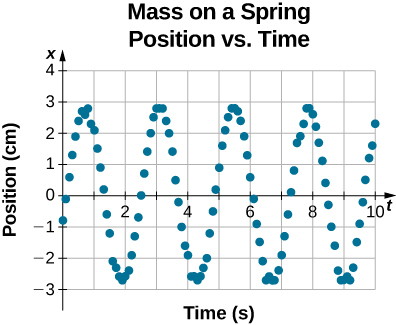The data in (Figure) can still be modeled with a periodic function, like a cosine function, but the function is shifted to the right. This shift is known as a phase shift and is usually represented by the Greek letter phi $\left(\varphi \right)$. The equation of the position as a function of time for a block on a spring becomes

$x\left(t\right)=A\phantom{\rule{0.1em}{0ex}}\text{cos}\left(\omega t+\varphi \right).$

This is the generalized equation for SHM where t is the time measured in seconds, $\omega$ is the angular frequency with units of inverse seconds, A is the amplitude measured in meters or centimeters, and $\varphi$ is the phase shift measured in radians ((Figure)). It should be noted that because sine and cosine functions differ only by a phase shift, this motion could be modeled using either the cosine or sine function.

(a) A cosine function. (b) A cosine function shifted to the right by an angle $\varphi$. The angle $\varphi$ is known as the phase shift of the function.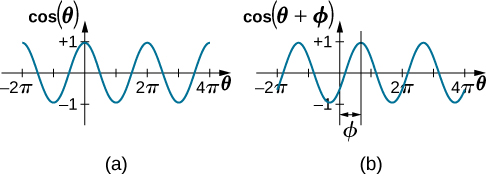The velocity of the mass on a spring, oscillating in SHM, can be found by taking the derivative of the position equation:

$v\left(t\right)=\frac{dx}{dt}=\frac{d}{dt}\left(A\text{cos}\left(\omega t+\varphi \right)\right)=\text{−}A\omega \text{sin}\left(\omega t+\phi \right)=\text{−}{v}_{\text{max}}\text{sin}\left(\omega t+\varphi \right).$

Because the sine function oscillates between –1 and +1, the maximum velocity is the amplitude times the angular frequency, ${v}_{\text{max}}=A\omega$. The maximum velocity occurs at the equilibrium position $\left(x=0\right)$ when the mass is moving toward $x=+A$. The maximum velocity in the negative direction is attained at the equilibrium position $\left(x=0\right)$ when the mass is moving toward $x=\text{−}A$ and is equal to $\text{−}{v}_{\text{max}}$.

The acceleration of the mass on the spring can be found by taking the time derivative of the velocity:

$a\left(t\right)=\frac{dv}{dt}=\frac{d}{dt}\left(\text{−}A\omega \text{sin}\left(\omega t+\varphi \right)\right)=\text{−}A{\omega }^{2}\text{cos}\left(\omega t+\phi \right)=\text{−}{a}_{\text{max}}\text{cos}\left(\omega t+\varphi \right).$

The maximum acceleration is ${a}_{\text{max}}=A{\omega }^{2}$. The maximum acceleration occurs at the position$\left(x=\text{−}A\right)$, and the acceleration at the position $\left(x=\text{−}A\right)$ and is equal to $\text{−}{a}_{\text{max}}$.

### Summary of Equations of Motion for SHM

In summary, the oscillatory motion of a block on a spring can be modeled with the following equations of motion:

$x\left(t\right)=A\text{cos}\left(\omega t+\varphi \right)$
$v\left(t\right)=\text{−}{v}_{\text{max}}\text{sin}\left(\omega t+\varphi \right)$
$a\left(t\right)=\text{−}{a}_{\text{max}}\text{cos}\left(\omega t+\varphi \right)$
${x}_{\text{max}}=A$
${v}_{\text{max}}=A\omega$
${a}_{\text{max}}=A{\omega }^{2}.$

Here, A is the amplitude of the motion, T is the period, $\varphi$ is the phase shift, and $\omega =\frac{2\pi }{T}=2\pi f$ is the angular frequency of the motion of the block.

Determining the Equations of Motion for a Block and a Spring
A 2.00-kg block is placed on a frictionless surface. A spring with a force constant of $k=32.00\phantom{\rule{0.2em}{0ex}}\text{N}\text{/}\text{m}$ is attached to the block, and the opposite end of the spring is attached to the wall. The spring can be compressed or extended. The equilibrium position is marked as $x=0.00\phantom{\rule{0.2em}{0ex}}\text{m}\text{.}$

Work is done on the block, pulling it out to $x=+0.02\phantom{\rule{0.2em}{0ex}}\text{m}\text{.}$ The block is released from rest and oscillates between $x=+0.02\phantom{\rule{0.2em}{0ex}}\text{m}$ and $x=-0.02\phantom{\rule{0.2em}{0ex}}\text{m}\text{.}$ The period of the motion is 1.57 s. Determine the equations of motion.

Strategy
We first find the angular frequency. The phase shift is zero, $\varphi =0.00\phantom{\rule{0.2em}{0ex}}\text{rad,}$ because the block is released from rest at $x=A=+0.02\phantom{\rule{0.2em}{0ex}}\text{m}\text{.}$ Once the angular frequency is found, we can determine the maximum velocity and maximum acceleration.

Solution
The angular frequency can be found and used to find the maximum velocity and maximum acceleration:

$\begin{array}{ccc}\hfill \omega & =\hfill & \frac{2\pi }{1.57\phantom{\rule{0.2em}{0ex}}\text{s}}=4.00\phantom{\rule{0.2em}{0ex}}{\text{s}}^{-1};\hfill \\ \hfill {v}_{\text{max}}& =\hfill & A\omega =0.02\text{m}\left(4.00\phantom{\rule{0.2em}{0ex}}{\text{s}}^{-1}\right)=0.08\phantom{\rule{0.2em}{0ex}}\text{m/s;}\hfill \\ \hfill {a}_{\text{max}}& =\hfill & A{\omega }^{2}=0.02\phantom{\rule{0.2em}{0ex}}\text{m}{\left(4.00\phantom{\rule{0.2em}{0ex}}{\text{s}}^{-1}\right)}^{2}=0.32{\phantom{\rule{0.2em}{0ex}}\text{m/s}}^{2}.\hfill \end{array}$

All that is left is to fill in the equations of motion:

$\begin{array}{ccc}\hfill x\left(t\right)& =\hfill & A\phantom{\rule{0.1em}{0ex}}\text{cos}\left(\omega t+\varphi \right)=\left(0.02\phantom{\rule{0.2em}{0ex}}\text{m}\right)\text{cos}\left(4.00\phantom{\rule{0.2em}{0ex}}{\text{s}}^{-1}t\right);\hfill \\ \hfill v\left(t\right)& =\hfill & \text{−}{v}_{\text{max}}\text{sin}\left(\omega t+\varphi \right)=\left(-0.08\phantom{\rule{0.2em}{0ex}}\text{m/s}\right)\text{sin}\left(4.00\phantom{\rule{0.2em}{0ex}}{\text{s}}^{-1}t\right);\hfill \\ a\left(t\right)\hfill & =\hfill & \text{−}{a}_{\text{max}}\text{cos}\left(\omega t+\varphi \right)=\left(-0.32\phantom{\rule{0.2em}{0ex}}{\text{m/s}}^{2}\right)\text{cos}\left(4.00\phantom{\rule{0.2em}{0ex}}{\text{s}}^{-1}t\right).\hfill \end{array}$

Significance
The position, velocity, and acceleration can be found for any time. It is important to remember that when using these equations, your calculator must be in radians mode.

### The Period and Frequency of a Mass on a Spring

One interesting characteristic of the SHM of an object attached to a spring is that the angular frequency, and therefore the period and frequency of the motion, depend on only the mass and the force constant, and not on other factors such as the amplitude of the motion. We can use the equations of motion and Newton’s second law $\left({\stackrel{\to }{F}}_{\text{net}}=m\stackrel{\to }{a}\right)$ to find equations for the angular frequency, frequency, and period.

Consider the block on a spring on a frictionless surface. There are three forces on the mass: the weight, the normal force, and the force due to the spring. The only two forces that act perpendicular to the surface are the weight and the normal force, which have equal magnitudes and opposite directions, and thus sum to zero. The only force that acts parallel to the surface is the force due to the spring, so the net force must be equal to the force of the spring:

$\begin{array}{ccc}\hfill {F}_{x}& =\hfill & \text{−}kx;\hfill \\ \\ \hfill ma& =\hfill & \text{−}kx;\hfill \\ \\ \\ \hfill m\frac{{d}^{2}x}{d{t}^{2}}& =\hfill & \text{−}kx;\hfill \\ \hfill \frac{{d}^{2}x}{d{t}^{2}}& =\hfill & -\frac{k}{m}x.\hfill \end{array}$

Substituting the equations of motion for x and a gives us

$\text{−}A{\omega }^{2}\text{cos}\left(\omega t+\varphi \right)=-\frac{k}{m}A\text{cos}\left(\omega t+\varphi \right).$

Cancelling out like terms and solving for the angular frequency yields

$\omega =\sqrt{\frac{k}{m}}.$

The angular frequency depends only on the force constant and the mass, and not the amplitude. The angular frequency is defined as $\omega =2\pi \text{/}T,$ which yields an equation for the period of the motion:

$T=2\pi \sqrt{\frac{m}{k}}.$

The period also depends only on the mass and the force constant. The greater the mass, the longer the period. The stiffer the spring, the shorter the period. The frequency is

$f=\frac{1}{T}=\frac{1}{2\pi }\sqrt{\frac{k}{m}}.$

### Vertical Motion and a Horizontal Spring

When a spring is hung vertically and a block is attached and set in motion, the block oscillates in SHM. In this case, there is no normal force, and the net effect of the force of gravity is to change the equilibrium position. Consider (Figure). Two forces act on the block: the weight and the force of the spring. The weight is constant and the force of the spring changes as the length of the spring changes.

A spring is hung from the ceiling. When a block is attached, the block is at the equilibrium position where the weight of the block is equal to the force of the spring. (a) The spring is hung from the ceiling and the equilibrium position is marked as ${y}_{o}$. (b) A mass is attached to the spring and a new equilibrium position is reached (${y}_{1}={y}_{o}-\text{Δ}y$) when the force provided by the spring equals the weight of the mass. (c) The free-body diagram of the mass shows the two forces acting on the mass: the weight and the force of the spring.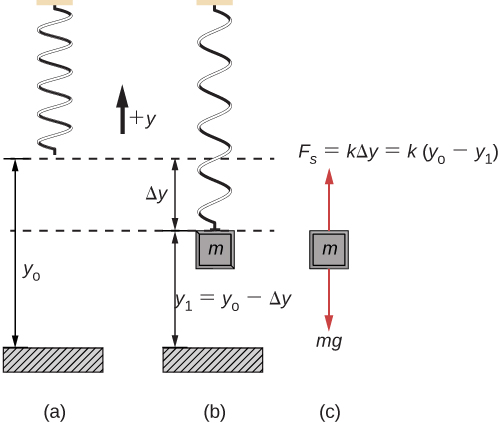When the block reaches the equilibrium position, as seen in (Figure), the force of the spring equals the weight of the block, ${F}_{\text{net}}={F}_{\text{s}}-mg=0$, where

$\text{−}k\left(\text{−}\text{Δ}y\right)=mg.$

From the figure, the change in the position is $\text{Δ}y={y}_{0}-{y}_{1}$ and since $\text{−}k\left(\text{−}\text{Δ}y\right)=mg$, we have

$k\left({y}_{0}-{y}_{1}\right)-mg=0.$

If the block is displaced and released, it will oscillate around the new equilibrium position. As shown in (Figure), if the position of the block is recorded as a function of time, the recording is a periodic function.

If the block is displaced to a position y, the net force becomes ${F}_{\text{net}}=k\left(y-{y}_{0}\right)-mg=0$. But we found that at the equilibrium position, $mg=k\text{Δ}y=k{y}_{0}-k{y}_{1}$. Substituting for the weight in the equation yields

${F}_{\text{net}}=ky-k{y}_{0}-\left(k{y}_{0}-k{y}_{1}\right)=\text{−}k\left(y-{y}_{1}\right).$

Recall that ${y}_{1}$ is just the equilibrium position and any position can be set to be the point $y=0.00\text{m}\text{.}$ So let’s set ${y}_{1}$ to $y=0.00\phantom{\rule{0.2em}{0ex}}\text{m}\text{.}$ The net force then becomes

$\begin{array}{ccc}\hfill {F}_{\text{net}}& =\hfill & \text{−}ky;\hfill \\ \\ \\ \hfill m\frac{{d}^{2}y}{d{t}^{2}}& =\hfill & \text{−}ky.\hfill \end{array}$

This is just what we found previously for a horizontally sliding mass on a spring. The constant force of gravity only served to shift the equilibrium location of the mass. Therefore, the solution should be the same form as for a block on a horizontal spring, $y\left(t\right)=A\text{cos}\left(\omega t+\varphi \right).$ The equations for the velocity and the acceleration also have the same form as for the horizontal case. Note that the inclusion of the phase shift means that the motion can actually be modeled using either a cosine or a sine function, since these two functions only differ by a phase shift.

Graphs of y(t), v(t), and a(t) versus t for the motion of an object on a vertical spring. The net force on the object can be described by Hooke’s law, so the object undergoes SHM. Note that the initial position has the vertical displacement at its maximum value A; v is initially zero and then negative as the object moves down; the initial acceleration is negative, back toward the equilibrium position and becomes zero at that point.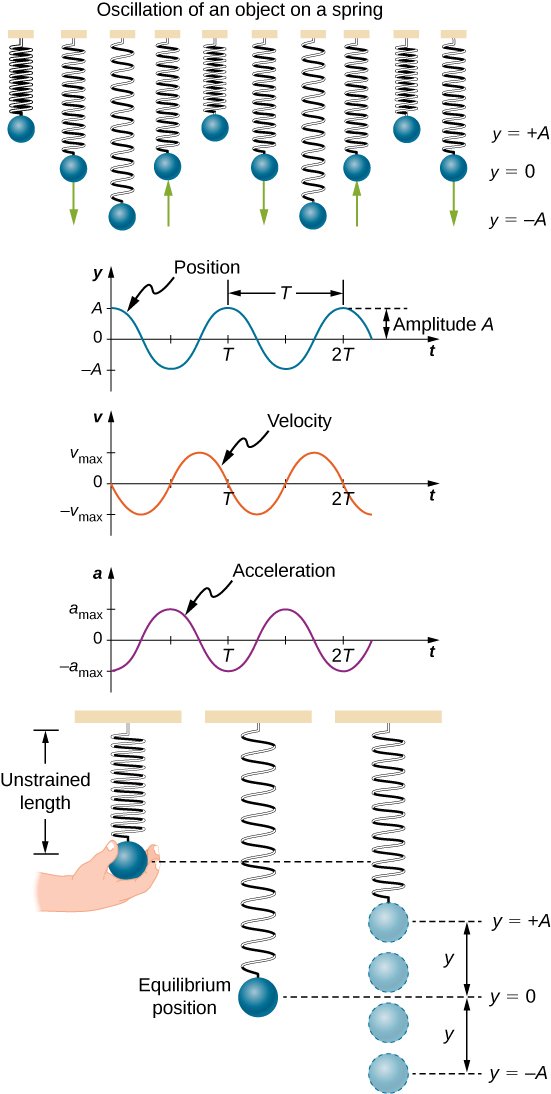### Summary

• Periodic motion is a repeating oscillation. The time for one oscillation is the period T and the number of oscillations per unit time is the frequency f. These quantities are related by $f=\frac{1}{T}$.
• Simple harmonic motion (SHM) is oscillatory motion for a system where the restoring force is proportional to the displacement and acts in the direction opposite to the displacement.
• Maximum displacement is the amplitude A. The angular frequency $\omega$, period T, and frequency f of a simple harmonic oscillator are given by $\omega =\sqrt{\frac{k}{m}}$, $T=2\pi \sqrt{\frac{m}{k}},\phantom{\rule{0.2em}{0ex}}\text{and}\phantom{\rule{0.2em}{0ex}}f=\frac{1}{2\pi }\sqrt{\frac{k}{m}}$, where m is the mass of the system and k is the force constant.
• Displacement as a function of time in SHM is given by$x\left(t\right)=A\phantom{\rule{0.2em}{0ex}}\text{cos}\left(\frac{2\pi }{T}t+\varphi \right)=A\text{cos}\left(\omega t+\varphi \right)$.
• The velocity is given by $v\left(t\right)=\text{−}A\omega \text{sin}\left(\omega t+\varphi \right)=\text{−}{v}_{\text{max}}\text{sin}\left(\omega t+\varphi \right),\phantom{\rule{0.2em}{0ex}}\text{where}\phantom{\rule{0.2em}{0ex}}{\text{v}}_{\text{max}}=A\omega =A\sqrt{\frac{k}{m}}$.
• The acceleration is $a\left(t\right)=\text{−}A{\omega }^{2}\text{cos}\left(\omega t+\varphi \right)=\text{−}{a}_{\text{max}}\text{cos}\left(\omega t+\varphi \right)$, where ${a}_{\text{max}}=A{\omega }^{2}=A\frac{k}{m}$.

### Conceptual Questions

What conditions must be met to produce SHM?

The restoring force must be proportional to the displacement and act opposite to the direction of motion with no drag forces or friction. The frequency of oscillation does not depend on the amplitude.

(a) If frequency is not constant for some oscillation, can the oscillation be SHM? (b) Can you think of any examples of harmonic motion where the frequency may depend on the amplitude?

Give an example of a simple harmonic oscillator, specifically noting how its frequency is independent of amplitude.

Examples: Mass attached to a spring on a frictionless table, a mass hanging from a string, a simple pendulum with a small amplitude of motion. All of these examples have frequencies of oscillation that are independent of amplitude.

Explain why you expect an object made of a stiff material to vibrate at a higher frequency than a similar object made of a more pliable material.

As you pass a freight truck with a trailer on a highway, you notice that its trailer is bouncing up and down slowly. Is it more likely that the trailer is heavily loaded or nearly empty? Explain your answer.

Since the frequency is proportional to the square root of the force constant and inversely proportional to the square root of the mass, it is likely that the truck is heavily loaded, since the force constant would be the same whether the truck is empty or heavily loaded.

Some people modify cars to be much closer to the ground than when manufactured. Should they install stiffer springs? Explain your answer.

### Problems

Prove that using $x\left(t\right)=A\text{sin}\left(\omega t+\varphi \right)$ will produce the same results for the period for the oscillations of a mass and a spring. Why do you think the cosine function was chosen?

Proof

What is the period of 60.0 Hz of electrical power?

If your heart rate is 150 beats per minute during strenuous exercise, what is the time per beat in units of seconds?

0.400 s/beat

Find the frequency of a tuning fork that takes $2.50\phantom{\rule{0.2em}{0ex}}×\phantom{\rule{0.2em}{0ex}}{10}^{-3}\text{s}$ to complete one oscillation.

A stroboscope is set to flash every $8.00\phantom{\rule{0.2em}{0ex}}×\phantom{\rule{0.2em}{0ex}}{10}^{-5}\text{s}$. What is the frequency of the flashes?

12,500 Hz

A tire has a tread pattern with a crevice every 2.00 cm. Each crevice makes a single vibration as the tire moves. What is the frequency of these vibrations if the car moves at 30.0 m/s?

Each piston of an engine makes a sharp sound every other revolution of the engine. (a) How fast is a race car going if its eight-cylinder engine emits a sound of frequency 750 Hz, given that the engine makes 2000 revolutions per kilometer? (b) At how many revolutions per minute is the engine rotating?

a. 340 km/hr; b. $11.3\phantom{\rule{0.2em}{0ex}}×\phantom{\rule{0.2em}{0ex}}{10}^{3}$ rev/min

A type of cuckoo clock keeps time by having a mass bouncing on a spring, usually something cute like a cherub in a chair. What force constant is needed to produce a period of 0.500 s for a 0.0150-kg mass?

A mass ${m}_{0}$ is attached to a spring and hung vertically. The mass is raised a short distance in the vertical direction and released. The mass oscillates with a frequency ${f}_{0}$. If the mass is replaced with a mass nine times as large, and the experiment was repeated, what would be the frequency of the oscillations in terms of ${f}_{0}$
?

$f=\frac{1}{3}{f}_{0}$

A 0.500-kg mass suspended from a spring oscillates with a period of 1.50 s. How much mass must be added to the object to change the period to 2.00 s?

By how much leeway (both percentage and mass) would you have in the selection of the mass of the object in the previous problem if you did not wish the new period to be greater than 2.01 s or less than 1.99 s?

0.009 kg; 2%

### Glossary

amplitude (A)
maximum displacement from the equilibrium position of an object oscillating around the equilibrium position
equilibrium position
position where the spring is neither stretched nor compressed
force constant (k)
characteristic of a spring which is defined as the ratio of the force applied to the spring to the displacement caused by the force
frequency (f)
number of events per unit of time
oscillation
single fluctuation of a quantity, or repeated and regular fluctuations of a quantity, between two extreme values around an equilibrium or average value
periodic motion
motion that repeats itself at regular time intervals
period (T)
time taken to complete one oscillation
phase shift
angle, in radians, that is used in a cosine or sine function to shift the function left or right, used to match up the function with the initial conditions of data
simple harmonic motion (SHM)
oscillatory motion in a system where the restoring force is proportional to the displacement, which acts in the direction opposite to the displacement
simple harmonic oscillator
a device that oscillates in SHM where the restoring force is proportional to the displacement and acts in the direction opposite to the displacement